# Le Chateliers Principle

## Le Chateliers Principle

This principle is based on the fundamentals of a stable chemical equilibrium i.e. when a chemical equilibrium is disturbed, it shifts in a direction to nullify the effect of change produced and a new equilibrium state is reached which may or may not have the same value of equilibrium constant. It states that “a chemical reaction at equilibrium is a stable equilibrium and always reverts back to its equilibrium state when disturbed from this state by any external factor such as, concentration, pressure, temperature etc”.

This statement will be explained with the help of following illustration.

Let us consider the equilibrium, 2NH3(g)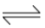N2(g) + 3H2(g)

Let the moles of N2, H2 and NH3 at equilibrium be a, b and c moles respectively. Since the reaction is at equilibrium,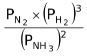= KP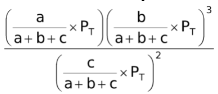= KP

where PT is the total pressure of the system.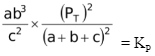= KP

Since PT =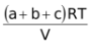( assuming all gases to be ideal)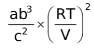= KP….(1)

Now, let us examine the effect of change in certain parameters such as number of moles, pressure, temperature, addition of inert gas etc.

(i) If we increase a or b, the left hand side expression becomes QP ( as it is disturbed from equilibrium) and we can see that QP > KP. The reaction therefore moves backward to make QP = KP.

If we increase c, QP < KP and the reaction has to move forward to revert back to equilibrium.

Thus generalization about effect of change of concentration of reactants or products is

(a) When more reactant is added to, or some product is removed from an equilibrium mixture, the net reaction occurs in the forward direction (as Q < K) to give a new equilibrium, and more products are produced.

(b) When more product is added to, or some reactant is removed from an equilibrium mixture, the net reaction moves in the reverse direction (as Q > K) to give a new equilibrium, and more reactants are produced.

(ii) If we increase the volume of the container (which amounts to decreasing the pressure),
QP < KP and the reaction moves forward to attain equilibrium. Thus, it can be generalized that

If the pressure is decreased by increasing the volume of a reaction mixture, the reaction shifts in the direction of more moles of gas while if the pressure is increased by decreasing the volume of a reaction mixture, the reaction shifts in the direction of fewer moles of gas.

(iii) Let us now introduce non – reacting gas (inert gas) to the system at constant volume. Since V remains fixed, a, b and c also remains fixed and RT is any way a constant, thus we see that QP = KP. Therefore, the reaction does not move at all. Thus, generalization is

When the addition of inert gas is carried out at constant volume, the equilibrium remains unaffected for reactions whether they have Δn = 0 or Δn ≠ 0.

(iv) If we add the non – reacting gas (inert gas) at constant pressure (which means increase in the volume of vessel) therefore QP < KP and the reaction move forward to attain equilibrium.

Thus, it can be generalized that

(a) The addition of an inert gas at constant pressure to an equilibrium, whose Δn ≠ 0, the equilibrium shifts in the direction of greater number of moles.

(b) When Δn = 0, there will be no effect of addition of inert gas at constant pressure on chemical equilibrium reaction (as V would not exist in the expression of Kp).

(v) You can clearly see the importance of forming an equation like equation (1). It helps you to analyse the equilibrium reaction quantitatively without dwelling unnecessarily into theoretical predictions. Moreover, this equation also analyses the effect of changing two or more parameters simultaneously. For example, if we double the volume of the vessel and increase the moles of nitrogen four times its initial value, QP would still be equal to KP and there would be no effect on the reaction.

(vi) Now, let us analyse the effect of temperature on the equilibrium reaction. If we increase the temperature of the reaction, the left hand side increases (QP) and therefore does it mean that the reaction goes backward (since QP > KP)?. Does this also mean that if the number of moles of reactant and product gases are equal, no change in the reaction is observed on changing temperature (as T would not exist in the expression of Kp). The answer to these questions is No. This is because KP also changes with temperature. Therefore, we are required to know the effect of temperature on both QP and KP to decide the fate of the equilibrium reaction.

As per Von’t Hoff temperature isotherm

log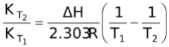For an exothermic reaction , ΔH would be negative. If we increase the temperature of the system (T2 >T1), the right hand side of the above equation becomes negative.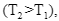, that is, the equilibrium constant at the higher temperature would be less than that at the lower temperature.

Now let us come back to our question once again. Will the reaction go in forward or reverse reaction? Before answering this, we must first encounter another problem. If temperature is increased, the new KP would either increase or decrease or may remain same. Let us say it increases (as the equilibrium reaction is endothermic)

Now, QP can also increase, decrease or remain unchanged. If KP increases and QP decreases, than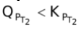, therefore the reaction moves forward. If KP increase and QP remains same, then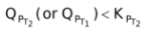. Again, the reaction moves forward. Let us see what would happen if KP increases and QP also increases. Will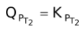or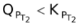or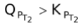? This can be answered by simply looking at the dependence of QP and KP on temperature. You can see from the equation (6) that KP depends on temperature exponentially, while Q’s dependence on T would be either to the power x or y or z or some other number. Therefore the variation in KP with increasing temperature would be more than in QP.

∴ KP would still be greater than QP and the reaction moves forward again.

Therefore, to see the temperature effect, we need to look at KP only. If it increases the reaction moves forward, if it decreases, reaction moves backward and if it remains fixed, then no change occurs at all. Thus the effect of change of temperature can be generalized as

(a) If ΔH is +ve (endothermic) an increase in temperature (T2 > T1) will make K2 > K1, i.e. the reaction goes more towards the forward direction and vice-versa.

(b) If ΔH is −ve (exothermic) an increase in temperature (T2 > T1), will take K2 < K1 i.e., the reaction goes in the reverse direction.

Application of Le Chatelier principle to physical equilibria

### (1) Effect of Pressure on Melting Points

(a) When volume of solid decreases on melting i.e., in liquid state volume is lesser, then by increasing pressure on such solids the melting point will go down, because at high pressure melting is facilitated e.g. Ice, diamond, carborundum (SiC) etc.

Solid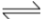Liquid

(b) When volume increases on melting then by increasing pressure, the above equilibria will shift in the reverse direction, hence process of melting will be lowered and more heat is required thus melting point will rise e.g. Iron, Copper, NH4Cl, NaCl etc.

SolidLiquid

### (2) Effect of Pressure on Boiling Point

When pressure is raised then condensation of vapour into liquid take place, thus vapour pressure will decrease. Now more heat is required to equate vapour pressure with atmospheric pressure, hence boiling point will increase.